# Multiplying Decimals by Whole Numbers

##By SmithMathAcademy

Multiplying Decimals by Whole Numbers# Multiplying Fractions by Whole Numbers

##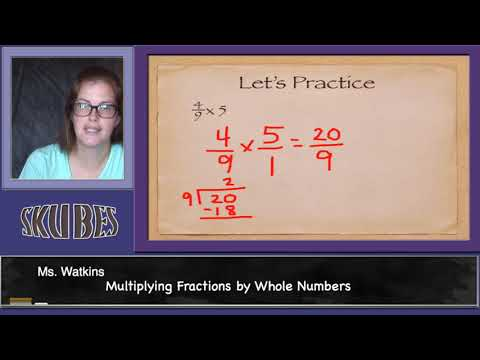By Skubes ed

Understand a multiple of a/b as a multiple of 1/b, and use this understanding to multiply a fraction by a whole number.# Multiplying Fractions by Whole Numbers 9-9

##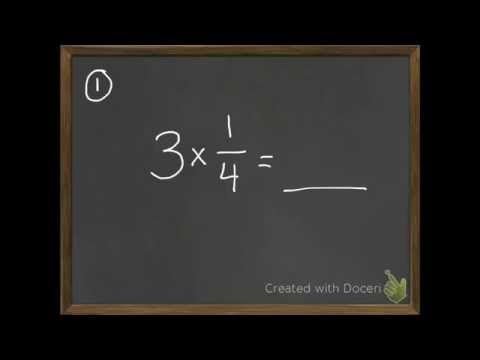By David Horan

multiply a fraction with a whole number# Multiplying Fractions by Whole Numbers 9-9

##By David Horan

multiply a fraction with a whole number.# Multiplying fractions and whole numbers: mixed number answer

##By Khan Academy

This video lecture series on Developmental Math by Khan Academy provides developmental math examples from the Monterey Institute. These start pretty basic and would prepare a student for the Worked Examples in Algebra course lectures.....# Multiply fractions by whole numbers

##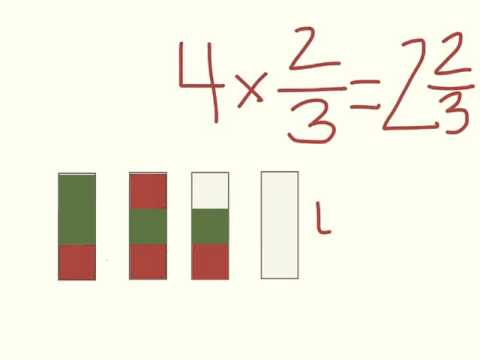By Gregory Asplund

Mutliplication of whole numbers# Multiplying Whole Numbers with Fractions

##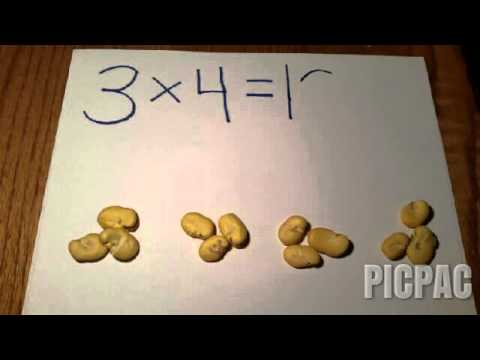By

Understand a fraction a/b as a multiple of 1/b# Multiplying fractions by whole numbers 4.NF.B.4 4.NF.4

##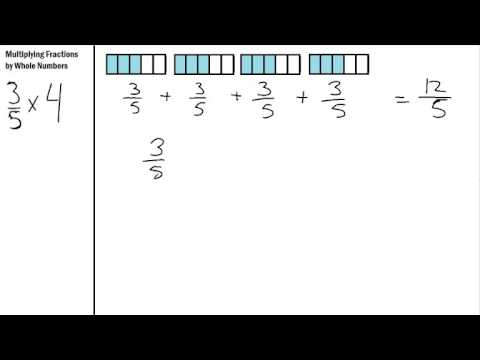By S Hart

Multiplying Fractions by Whole numbers using repeated addition# Conceptual understanding of multiplying fractions and whole numbers | Khan Academy

##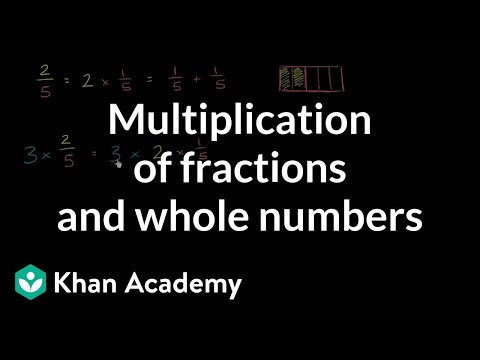By Khan Academy

Here we have a nice visual explanation of how a mixed number is really a multiplication of a fraction and a whole number.# Multiplying fractions by whole numbers word problem | Math | 4th grade | Khan Academy

##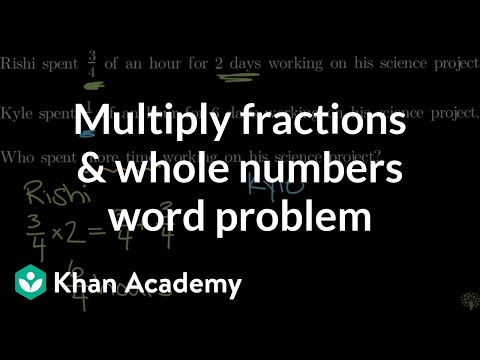By Khan Academy

solves a word problem that involves multiplying a fraction by a whole number# Multiplying Fractions by Whole Numbers

##By Skubes ed

Understand a multiple of a/b as a multiple of 1/b, and use this understanding to multiply a fraction by a whole number# Multiplying fractions by whole numbers word problem | Math | 4th grade | Khan Academy

##By KhanAcademy

solves a word problem that involves multiplying a fraction by a whole number# Multiplying Fractions by Whole Numbers in Word Problems

##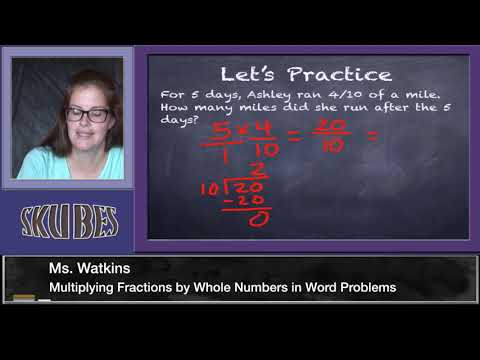By Skubes ed

Solve real world problems involving multiplication of fractions and mixed numbers, e.g., by using visual fraction models or equations to represent the problem# Finding Products of Polynomials

##By gdawgrapper

Finding Products of Polynomials# Grade 5 Math - Multiply Decimals 1

##By Lumos Learning

Using the Lumos Study Programs, parents and educators can reinforce the classroom learning experience for children and help them succeed at school and on the standardized tests. Lumos books, dvd, eLearning and tutoring are used by leading schools, libraries and thousands of parents to supplement classroom learning and improve student achievement in the standardized tests.

PARENTS please visit LumosTestPrep.com to learn more.
EDUCATORS please visit LumosLearning.com to learn more# Visually understanding multiplying fractions and whole numbers

##By Khan Academy

Here we have a nice visual explanation of how a mixed number is really a multiplication of a fraction and a whole number.# Multiplying Whole Numbers Framing Video

##By eSparkLearningVideos

This video narrates a word problem involving multiplication.# Whole number and fraction multiplication concept | 4th grade | Khan Academy

##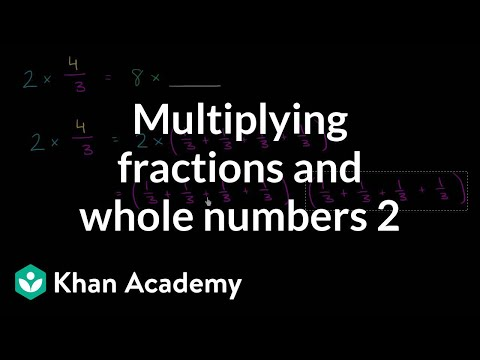By Khan Academy

A mixed number is simply a whole number/fraction multiplication problem. Let us explain.# Three-Digit by One Digit Multiplication with Partial Products: Grade 4

##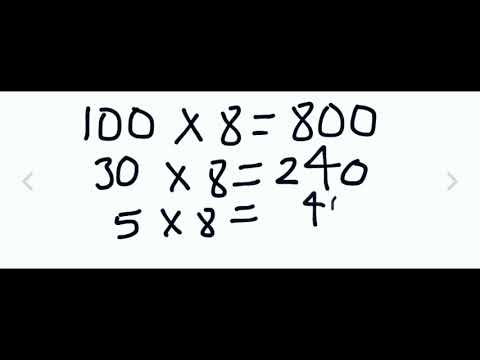By University Of Houstan Mathematics Education

Multiply a whole number of up to four digits by a one-digit whole number, and multiply two two-digit numbers# Box Multiplication: Partial Products

##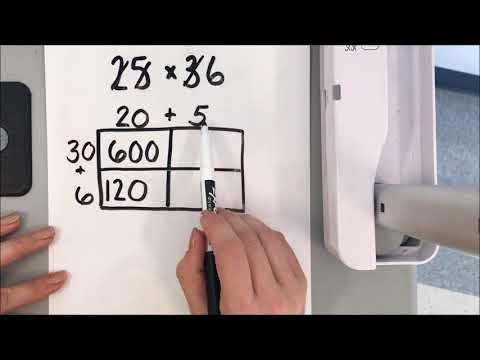By Erica Carmody

Multiply a whole number of up to four digits by a one-digit whole number, and multiply two two-digit numbers, using strategies based on place value and the properties of operations